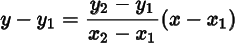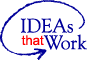# Best Practices for Authoring MathML in EPUB

## Important Update – Content has Moved

This work has moved over to the DAISY GitHub repository. Please follow these links for the latest recommendations. Main DAISY GitHub Repository, and the rendered view of this document.

## Introduction

MathML has been around for many years, but the support for MathML in web browsers and EBook reading systems was suboptimal. On the other hand reading Math is essential for students with disabilities for making progress in the field of science, technology, engineering and mathematics. Therefore we initialized a working group for developing the best practices for MathML which could enable users to read Math in wider range of EPUB reading environments. The working group came up with wide range of techniques and evaluated the reading experience in different combination of EPUB reading systems, operating systems, browsers and assistive technologies. Which was followed by selection of most widely supported techniques.

It is possible that the recommendations for EPUB 3 environments provided in this report are a little different from the recommendations for the web environment. Though most of EPUB 3 reading systems use components from web browsers, the reading systems tend to change and sometimes override the web browser functionality, which results in a somewhat different reading experience.

It is important to highlight that the reading systems, underlying browsers of reading systems and assistive technologies are making rapid progress for supporting MathML. In next 6 to 12 months we expect revolutionary positive change in MathML reading experience. Therefore it is very much possible that the recommendations of this report become out of date very soon. Due to this reason we would like to provide interim recommendations at this time. The readers of this report should consider the recommendations as temporary or interim, the reading environments will be tested again after some months for providing concrete recommendations.

## Evaluation Process

Step 1. Development of techniques for evaluation. The following techniques were developed:

1. PNG image with alt text: Very basic test in which PNG image of Math expression is used for visual users and description of math expression is placed in the alt text of the image for assistive technology.
2. Javascript workaround for image+alt text along with hidden MathML: By default an image of Math equation, described in alt text is shown. If java script is enabled then it places visually hidden MathML for assistive technology and removes the alt text of image to prevent duplicate reading.
3. Image+alt text along with hidden MathML, but no java script: The same as above test, but java script is not used to present hidden MathML and hide alt text. Therefore, assistive technology will come across both hidden MathML as well as image alt text.
4. HTML details based tests: The Math expression in image is shown along with alt text with description of expression. In addition, HTML details element is placed just after the image, and it contains MathML mark-up. Therefore image and alt text of expression will be always available, and if the reading system and assistive technology supports MathML, then user can open the HTML details to read MathML.
5. MathML mark-up with Image and alt text placed in annotation XML: This test mainly checks if annotation XML in MathML is supported by reading environments.
6. aria-label placed on parent element of MathML expression: It evaluates the behavior of aria-label in presence of MathML.
7. MathML using m: namespacing: Evaluates reading experience of MathML mark-up when namespace URL is not applied directly on the MathML expression.
8. MathML using the role="math": Evaluates reading experience when role="math" is used.
9. Read aloud feature reads alttext in MathML: Evaluates if the read aloud feature of the reading systems reads the alt text of MathML mark-up.

Step 2. The techniques were evaluated on different configurations of reading environments. The following reading systems, operating systems, browsers and assistive technologies combinations were used:

• Reading systems: Vital Source Bookshelf online, Vital Source Bookshelf desktop, Text Help (read & write), Redshelf, Thorium desktop, Dolphin Easyreader, Apple Books and Google Playbooks.
• Operating systems: Windows 10, Mac 10, iOS and Android.
• Browsers: Chrome, Firefox, Edge and Safari.
• Assistive technologies: JAWS, NVDA, Voiceover, Talkback.

## Future Prospects

With the efforts of Math refresh community group in W3C, Chrome has implemented MathML, which is now available in the test builds. It is expected that it will start shipping in main stream Chrome releases within 6 to 12 months. Microsoft Edge is also based on Chrome, this means that Edge would start supporting MathML almost simultaneously. The two main players in United States higher education, Vital Source and Redshelf have committed to improve MathML support in their reading systems in time frame of 3 to 6 months. All these developments will drastically improve MathML support in next one year.

## Analysis and Recommendations

Based on test results and future prospects, the following techniques are recommended as interim solution.

### Technique 1: Native MathML

Right now native MathML is supported by 7 of the tested 25 reading combinations. But looking at the future prospects, this would be the best way forward.

#### Code Example #1: Block MathML


<span id="block-mathml">
Some Text before the math equation
<math xmlns="http://www.w3.org/1998/Math/MathML" display="block" alttext="y minus y 1 equals StartFraction y 2 minus y 1 Over x 2 minus x 1 EndFraction left-parenthesis x minus x 1 right-parenthesis">
<mrow>
<mi>y</mi>
<mo>−</mo>
<msub>
<mi>y</mi>
<mn>1</mn>
</msub>
</mrow>
<mo>=</mo>
<mrow>
<mfrac>
<mrow>
<msub>
<mi>y</mi>
<mn>2</mn>
</msub>
<mo>−</mo>
<msub>
<mi>y</mi>
<mn>1</mn>
</msub>
</mrow>
<mrow>
<msub>
<mi>x</mi>
<mn>2</mn>
</msub>
<mo>−</mo>
<msub>
<mi>x</mi>
<mn>1</mn>
</msub>
</mrow>
</mfrac>
<mo>⁢<!--invisible times--></mo>
<mrow>
<mo>(</mo>
<mrow>
<mi>x</mi>
<mo>−</mo>
<msub>
<mi>x</mi>
<mn>1</mn>
</msub>
</mrow>
<mo>)</mo>
</mrow>
</mrow>
[/itex]
Some text after the equation.
</span>


#### Code Example #1 Preview: Block MathML

Some Text before the math equation $y minus y 1 equals StartFraction y 2 minus y 1 Over x 2 minus x 1 EndFraction left-parenthesis x minus x 1 right-parenthesis$ Some text after the equation.

#### Code Example #2: Inline MathML


<span id="inline-mathml">
Some Text before the math equation
<math xmlns="http://www.w3.org/1998/Math/MathML" display="inline" alttext="y minus y 1 equals StartFraction y 2 minus y 1 Over x 2 minus x 1 EndFraction left-parenthesis x minus x 1 right-parenthesis">
<mrow>
<mi>y</mi>
<mo>−</mo>
<msub>
<mi>y</mi>
<mn>1</mn>
</msub>
</mrow>
<mo>=</mo>
<mrow>
<mfrac>
<mrow>
<msub>
<mi>y</mi>
<mn>2</mn>
</msub>
<mo>−</mo>
<msub>
<mi>y</mi>
<mn>1</mn>
</msub>
</mrow>
<mrow>
<msub>
<mi>x</mi>
<mn>2</mn>
</msub>
<mo>−</mo>
<msub>
<mi>x</mi>
<mn>1</mn>
</msub>
</mrow>
</mfrac>
<mo>⁢<!--invisible times--></mo>
<mrow>
<mo>(</mo>
<mrow>
<mi>x</mi>
<mo>−</mo>
<msub>
<mi>x</mi>
<mn>1</mn>
</msub>
</mrow>
<mo>)</mo>
</mrow>
</mrow>
[/itex]
Some text after the equation.
</span>


#### Code Example #2 Preview: Inline MathML

Some Text before the math equation $y minus y 1 equals StartFraction y 2 minus y 1 Over x 2 minus x 1 EndFraction left-parenthesis x minus x 1 right-parenthesis$ Some text after the equation.

### Technique 2: Image with alt text followed by MathML placed in HTML details

The technique has the following caveats:

• It only works for block Math expressions.
• HTML details is also not so widely supported in reading combinations.
• The complexity of reading Math expression from image + alt text and then opening HTML details for reading each expression will result in considerable cognitive load, especially for people with cognitive disabilities.

#### Code Example #3: Image with alt text followed by MathML placed in HTML details


<img src="http://diagramcenter.org/wp-content/uploads/2020/09/epub-image-2pointform.png" alt="y minus y 1 equals StartFraction y 2 minus y 1 Over x 2 minus x 1 EndFraction left-parenthesis x minus x 1 right-parenthesis" aria-details="math-details1">

<details id="math-details1">
<summary>MathML Presentation</summary>
<math id="math1" xmlns="http://www.w3.org/1998/Math/MathML" display="block" alttext="(MathML alttext): y minus y sub 1 equals StartFraction y sub 2 minus y sub 1 Over x sub 2 minus x sub 1 EndFraction left-parenthesis x minus x sub 1 right-parenthesis">
<mrow>
<mi>y</mi>
<mo>−</mo>
<msub>
<mi>y</mi>
<mn>1</mn>
</msub>
</mrow>
<mo>=</mo>
<mrow>
<mfrac>
<mrow>
<msub>
<mi>y</mi>
<mn>2</mn>
</msub>
<mo>−</mo>
<msub>
<mi>y</mi>
<mn>1</mn>
</msub>
</mrow>
<mrow>
<msub>
<mi>x</mi>
<mn>2</mn>
</msub>
<mo>−</mo>
<msub>
<mi>x</mi>
<mn>1</mn>
</msub>
</mrow>
</mfrac>
<mo>⁢<!--invisible times--></mo>
<mrow>
<mo>(</mo>
<mrow>
<mi>x</mi>
<mo>−</mo>
<msub>
<mi>x</mi>
<mn>1</mn>
</msub>
</mrow>
<mo>)</mo>
</mrow>
</mrow>
[/itex]
</details>


#### Code Example #3 Preview: Image with alt text followed by MathML placed in HTML detailsMathML Presentation $(MathML alttext): y minus y sub 1 equals StartFraction y sub 2 minus y sub 1 Over x sub 2 minus x sub 1 EndFraction left-parenthesis x minus x sub 1 right-parenthesis$

### Technique 3: Image with alt text for inline expression

Many times simple Math expression is provided inline. It can be appropriately described by placing the description of the expression in the alt text of the image, therefore we can use image with alt text instead of inline MathML while the reading environments catch up. In order to preserve MathML mark-up for future use, the mark-up can be placed in comments instead of removing it.

#### Code Example #4: Image with alt text for inline expression


Text before math image
<img src="http://diagramcenter.org/wp-content/uploads/2020/09/epub-image-2pointform.png" alt="y minus y sub 1 equals StartFraction y sub 2 minus y sub 1 Over x sub 2 minus x sub 1 EndFraction left-parenthesis x minus x sub 1 right-parenthesis" aria-details="math-details1">
Text after math image.

<!-- Commented out MathML for future use
<math xmlns="http://www.w3.org/1998/Math/MathML" display="inline" alttext="y minus y 1 equals StartFraction y 2 minus y 1 Over x 2 minus x 1 EndFraction left-parenthesis x minus x 1 right-parenthesis">
<mrow>
<mi>y</mi>
<mo>−</mo>
<msub>
<mi>y</mi>
<mn>1</mn>
</msub>
</mrow>
<mo>=</mo>
<mrow>
<mfrac>
<mrow>
<msub>
<mi>y</mi>
<mn>2</mn>
</msub>
<mo>−</mo>
<msub>
<mi>y</mi>
<mn>1</mn>
</msub>
</mrow>
<mrow>
<msub>
<mi>x</mi>
<mn>2</mn>
</msub>
<mo>−</mo>
<msub>
<mi>x</mi>
<mn>1</mn>
</msub>
</mrow>
</mfrac>
<mo>⁢</mo>
<mrow>
<mo>(</mo>
<mrow>
<mi>x</mi>
<mo>−</mo>
<msub>
<mi>x</mi>
<mn>1</mn>
</msub>
</mrow>
<mo>)</mo>
</mrow>
</mrow>
[/itex]
End of commented out MathML-->


#### Code Example #4 Preview: Image with alt text for inline expression

Text before imageText after image.

Updated: 10/09/2020 Moved: 06/10/2022 (DAISY Github repo)The DIAGRAM Center is a Benetech initiative supported by the U.S. Department of Education, Office of Special Education Programs (Cooperative Agreement #H327B100001). Opinions expressed herein are those of the authors and do not necessarily represent the position of the U.S. Department of Education.